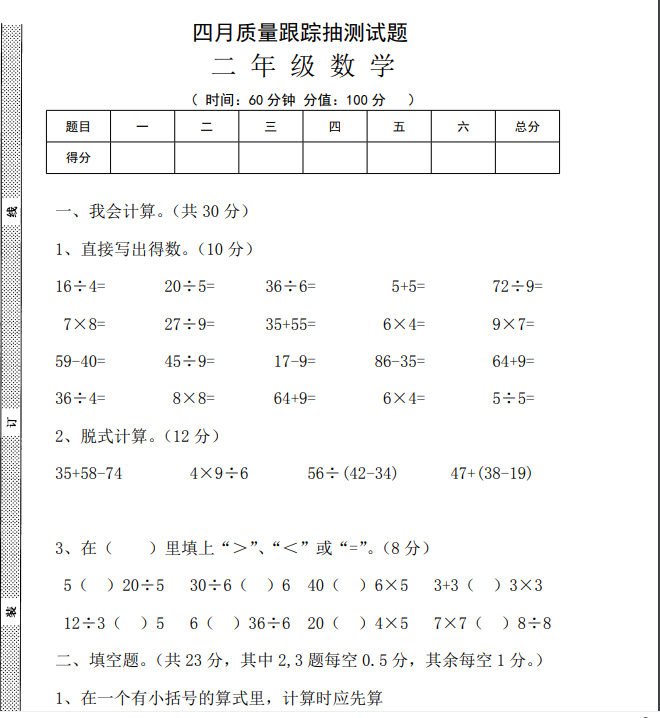1、直接写出得数。（10 分）
16÷4= 20÷5= 36÷6= 5+5= 72÷9=
7×8= 27÷9= 35+55= 6×4= 9×7=
59-40= 45÷9= 17-9= 86-35= 64+9=##### 评论信息